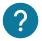# How does if/else work? How to use them in your program?

The if function allows you to execute code if the condition is true. The else will allow you to execute code if the condition is false. Finally the else if allows you to propose a second condition to check if the first if was not true. We will see step by step.

## If Condition

The if condition will allow you to test a part of your program with a condition: if this one is true, the code will be executed, if not, the program will go on.
``````if (condition) {
}``````

We see that the if function takes as parameter a condition which is boolean: either false or true.

We will see an example of a true condition:

``````if (7>5) {
}``````

By writing that 7 is greater than 5, the condition is true so the lines of code inside the brackets will be executed. By returning the condition, i.e. 7<5, the condition becomes false and therefore what is inside the brackets will not be executed.### How to write the condition?

The condition for the if can be hard to write, especially with all the operators that exist. We recommend our course on mathematical functions to have a complete table of all the operators that can be used on arduino!

## Else condition

The else is used when the if condition is false. It is an alternative to the if, if this one is not executed then the else will be.

Here is an example for the else:

``````if (condition) {
// If the condition is true
}

else {
// If the condition is false
}``````

Here is an example where the if condition is false so that the program executes the else:

``````if (7<5) {
Serial.println("if");// program not executed becasue the conditon is false
}

else {
Serial.println("else"); // Program executed
}``````

As you have seen, the else has no condition, so it will always be executed if the if is false. You may want to make another if to test a second condition. For that there is the “else if”.

## Else if condition

The “else if” allows to test a second condition after the first if. The “else if” like the simple if will be executed only if the condition is true. You can put as many “else if” as you want:

``````if (condition1) {
// program of the if
}
else if (condition2) {
// program of the first else if
}
else if (condition3) {
// the second else if
}
else {
//Code executed only if the conditions of if and elseif are false.
}``````

Here is an example:

``````if (4==3) { // false condition
Serial.println("if");// "if" program won't be executed
}
else if (4<2) { // false condition
Serial.println("first else if ");// the first "else if" won't be executed
}
else if (4>2) { // true conditon
Serial.println("second else if ");// the second "else if" will be executed
}
else {
Serial.println("else"); // this code won't be executed
}``````

Here as we can see, it is the second “else if” that will be executed because the conditions before it are false.

However, if you have a lot of “else if” to put, an alternative is the switch case.

## Bonus : Switch case

The switch case allows to test several conditions, making a cleaner code instead of using several if or else if. The switch case doesn’t replace in all cases the if and else if.

It uses a variable that contains an integer, a string or a boolean. Each cases is used to differentiate what the program must do if the variable is equal to the condition 1, or 2.  If the variable does not correspond to any case, then the code in default will be executed.

Here is an example:

``````int your_variable=1;
switch (your_variable) {
case 1:
Serial.println("your first condition"); // This case will be executed
break;
case 2:
Serial.println("your second condition"); This case won't be executed
break;
default:
Serial.println("default"); // Code will be executed only if the program didn't entered in any case
break;
}``````

In this example your variable will be compared to each case to know which code to execute. Here the value of “your_variable” is 1 so the executed code will be case 1.